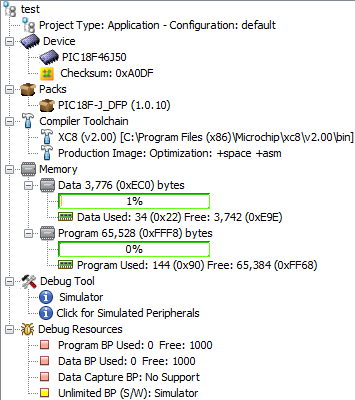## Thursday, 4 April 2019

### Optimising XC8 - Accessing Struct Members By Pointer

When passing struct data by pointer to a C function, the arrow operator "->" is typically used to dereference the pointer and access the value of a member.

On PIC 8-bit microcontrollers, using the MPLAB XC8 compiler, each access to a struct member from a pointer requires additional instructions to first deference the pointer before the value is made available. This may produce bloated code, resulting in a larger program and slower execution.

Version 2.00 of the XC8 compiler was used for this article. Optimization level was set to "1" (the highest level available in Free mode), and the target MCU was arbitrarily selected as PIC18F46J50.

Consider the following example:
```#include <xc.h>
#include <stdint.h>

typedef struct
{
uint8_t data;
} LARGESTRUCT_T;

static void modifyData(LARGESTRUCT_T *pdata)
{
pdata->data = 0;    // Access struct member directly.
pdata->data = 1;
pdata->data = 2;
pdata->data = 3;
pdata->data = 4;
pdata->data = 5;
pdata->data = 6;
pdata->data = 7;
pdata->data = 8;
pdata->data = 9;
pdata->data = 10;
pdata->data = 11;
pdata->data = 12;
pdata->data = 13;
pdata->data = 14;
pdata->data = 15;
}

static void useData(const LARGESTRUCT_T *pdata)
{
// Do something useful with data here.
}

void main(void)
{
LARGESTRUCT_T data;

for(;;)
{
modifyData(&data);
useData(&data);
}
}```
The space requirements for the above code:We are primarily concerned with the data and program memory used, which are 18 bytes of data memory, and 288 bytes of program memory.

Taking a look at the machine code produced by the compiler:
```;main.c: 12:     pdata->data = 1;
lfsr 2,1
movf modifyData@pdata,w,c
movf modifyData@pdata+1,w,c
movlw 1
movwf indf2,c
```
From the above snippet, we see each access to a member requires that its address be placed into the FSR2 register before a value is assigned to the memory address through the INDF2 register.

In some situations, instead of using a pointer to a struct, we can store a local copy of the data. The local copy is then modified as required, before the modified data is copied back to the pointer address:
```static void modifyData(LARGESTRUCT_T *pdata)
{
LARGESTRUCT_T localData = *pdata; // Store local copy of data.

localData.data = 0;            // Modify local copy.
localData.data = 1;
localData.data = 2;
localData.data = 3;
localData.data = 4;
localData.data = 5;
localData.data = 6;
localData.data = 7;
localData.data = 8;
localData.data = 9;
localData.data = 10;
localData.data = 11;
localData.data = 12;
localData.data = 13;
localData.data = 14;
localData.data = 15;

// Copy modified data back to pointer address.
*pdata = localData;
}
```
The machine code produced is greatly simplified:
```;main.c: 14:     localData.data = 1;
movlw 1
movwf modifyData@localData+1,c
```
Reviewing the reduced size of the code produced:The size of the program code produced was halved in this case. However, the amount of data memory has increased by the amount of data we have stored locally in the function (16 bytes). The good news is that as the size of data memory allocated is shared among functions with local storage as the compiler sees fit, you may see minimal increases depending on the rest of your application code.

In many of our projects, the trade off in added data memory requirements is worth the program memory and CPU cycles saved.